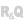HelpWLanguageWLanguage functionsControls, pages and windowsDiagramPresentationExampleProperties specific to the description of Diagram variablesSee alsoDiagramdiagActionAlloweddiagBorderdiagConnectionPointdiagConnectordiagGroupdiagHeaddiagImagediagLayoutdiagLibrarydiagOvaldiagPathdiagPointdiagPolygondiagRectanglediagSelectiondiagShapediagTextdiagWaypoint
• Properties specific to the description of Diagram variables
WINDEVWEBDEVWINDEV MobileOthersDiagram (Type of variable)
In french: Diagramme
The Diagram type is used to define all the advanced characteristics of a diagram that can be edited in the Diagram Editor control. The characteristics of this diagram can be defined and changed using different WLanguage properties.
Remark: For more details on the declaration of this type of variable and the use of WLanguage properties, see Declaring a variable.
Example
// Create a rectangle shape
MyShape is diagRectangle

// Set the dimensions, position and background color
MyShape.X = 100
MyShape.Y = 100
MyShape.Width = 350
MyShape.Height = 150
MyShape.Background.Color = PastelRed
MyShape.Comment = "Rectangle"

// Reference the displayed diagram
MyDiagram is Diagram <- DIAGEDT_MyDiagram

// Add the rectangle shape to the diagram

// Display the comment of the shape
Trace(MyDiagram.Shape.Comment)
// Move all shapes down
MyDiagram is Diagram <- DIAGEDT_MyDiagram.Value
FOR i = 1 TO MyDiagram.Shape.Count
Shape is diagShape
Shape <- MyDiagram.Shape[i]
Shape.Y += 10
END
Remarks

Properties specific to the description of Diagram variables

The following properties can be used to handle a diagram:
Property name Type used Effect BackgroundColor Integer Background color of the diagram. This property can correspond to: an RGB color (returned by RGB),an HSL color (returned by HSL),a WLanguage preset color.a variable of type Color. In this case, the opacity specified in the variable is taken into account. PageLayout diagLayout Diagram layout. This layout is used for printing the diagram. Real Spacing between gridlines. Shape Array of diagShape Array that contains the different shapes in the diagram. This property is read-only. Value String, Buffer, etc. Value of the diagram. This property can correspond to: a Diagram Editor control,the path of a Diagram file,a variable of type Buffer containing a diagram.
Minimum version required
• Version 27